Function Repository Resource:

# PersianImmortals

Simulate the self-organizing system of the Persian Immortals as described by Herodotus

Contributed by: Sander Huisman
 ResourceFunction["PersianImmortals"][] simulates 104 agents where the weakest agent is removed at every step and replaced by a new agent. ResourceFunction["PersianImmortals"][n] simulates 104 agents where n of the weakest agents are removed and replaced by new agents. ResourceFunction["PersianImmortals"][{n,m}] simulates 104 agents where n of the weakest agents and m random agents are removed. ResourceFunction["PersianImmortals"][…,pop] starts with an agent population pop. ResourceFunction["PersianImmortals"][…,pop,tspec] runs the simulation according to the time specification tspec. ResourceFunction["PersianImmortals"][…,pop,tspec,bins] uses the binning specification bins.

## Details and Options

The Persian Immortals were an elite army described by the Greek historian and geographer Herodotus while attacking Greece in 480-479 BCE under Xerxes's command. Their name refers to the fact that the size of the army was always kept constant at 10000 by immediately replacing every fallen warrior with a warrior from reserve troops. Because of this tactic, morale was kept high, and the army was actually getting stronger over time.
n determines how many of the weakest agents are removed:
 n Integer, remove the n weakest agents. dist Use the distribution dist to determine how many of the weakest agents are removed.
m determines how random agents are removed:
 m Integer, remove m agents randomly dist Use the distribution dist to determine how many random agents are removed {dist} Each agent gets a random threshold between 0 and its fitness. This threshold is then compared to a random attack given by dist. Fitter agents have a higher chance of survival.
pop describes the initial population:
 n Integer, n agents of fitnesses linearly chosen between 0 and 1. Automatic Use 104 agents of fitnesses linearly chosen between 0 and 1. {f1, f2,…} Use agents with fitness fi (should be between 0 and 1).
tspec describes the time for storing the output:
 n Integer, simulate n steps and save data from all timesteps. {tmin,tmax,Δt} Simulates for tmax steps but saves every Δt steps, starting from tmin. {{t1, t2,…}} Simulates until Max[ti] and saves each ti.
bins should be an integer that describes in how much bins the data should be binned. Fitnesses are assumed to be between 0 and 1.
PersianImmortals takes the following options:
 "Repetitions" Automatic number of times to simulate "NewFitness" Automatic fitness for new agents "Strengthening" Automatic specifies how the surviving agents strengthen over time
Possible settings for the option "Repetitions" include:
 Automatic perform a single simulation n perform n simulations and average the results
Possible settings for the option "NewFitness" include:
 Automatic generate agents with random fitnesses uniformly taken between 0 and 1 (UniformDistribution[0,1]) dist generate agents with random fitnesses taken from the distribution dist
Possible settings for the option "Strengthening" include:
 Automatic agents do not become stronger (equivalent to 0) val≤1 after each time step val is added to the fitness and clipped to[0,1] val>1 after each time step the fitness is multiplied by val and clipped to[0,1] Scaled[val] after each time step the fitness gets a fraction val closer to 1
ResourceFunction["PersianImmortals"] returns an Association with the following keys: "Population", "Repetitions", "BinDelimiters", "BinCenters", "BinCounts", "PDFs" and "CDFs".

## Examples

### Basic Examples (4)

Perform a simulation where, in each time-step, the weakest agent is replaced by a new random agent:

 In:=Out=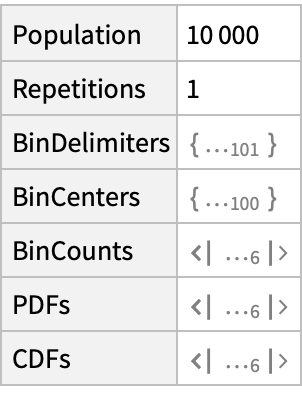Visualize the probability density function (PDF) for the fitness of the agents at various times:

 In:=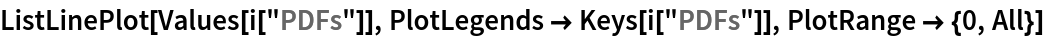Out=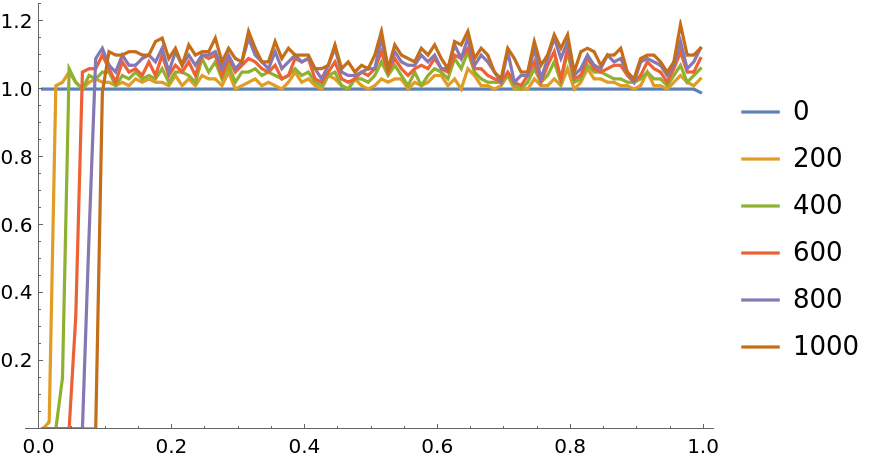At each step remove a random number between 1 and 10 of the weakest agents:

 In:=Out=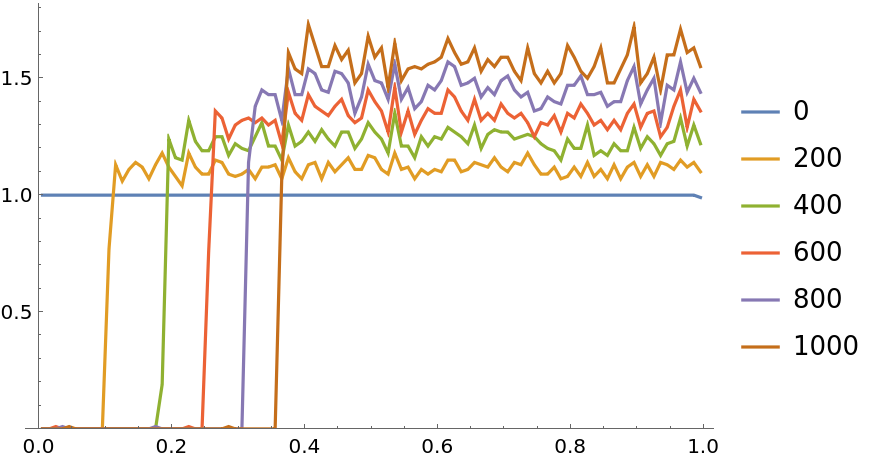At each step remove between 1 and 5 of the weakest agents and between 1 and 3 random agents:

 In:=Out=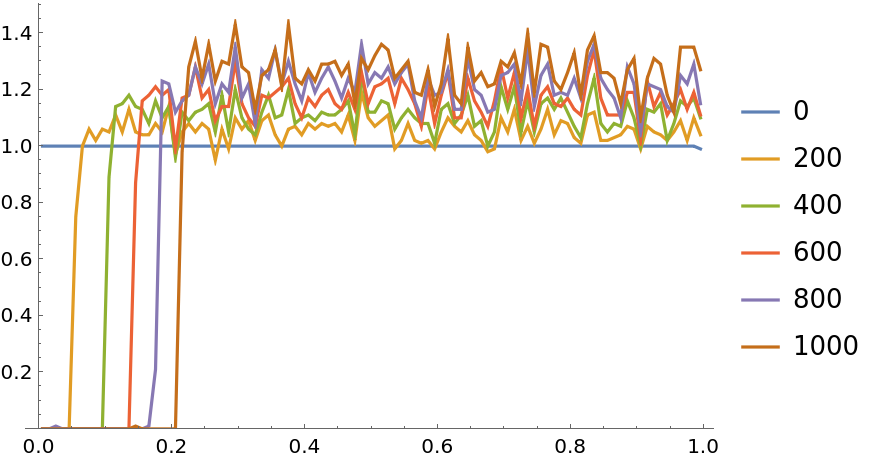Do not remove the weakest agents, but rather randomly remove agents based on a probabilistic distribution. Each agents creates a random threshold in the Range[0,fitness] and this is compared against a random number given by a distribution:

 In:=Out=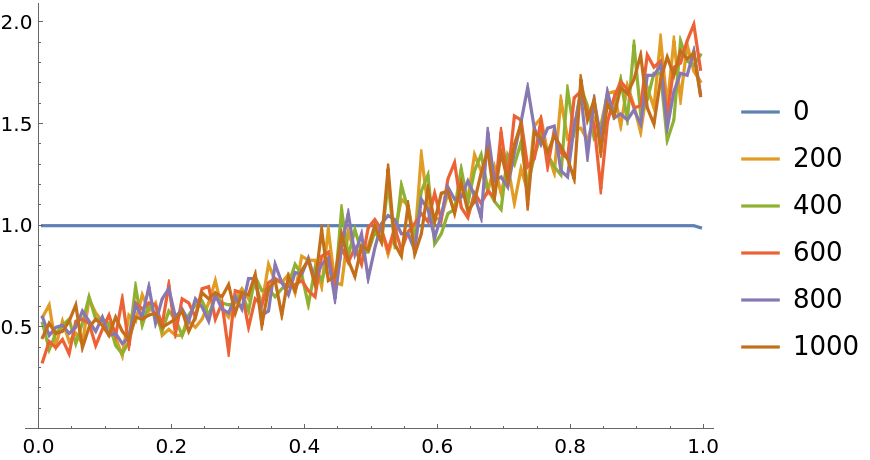### Scope (2)

Use an army of 100 agents:

 In:=Out=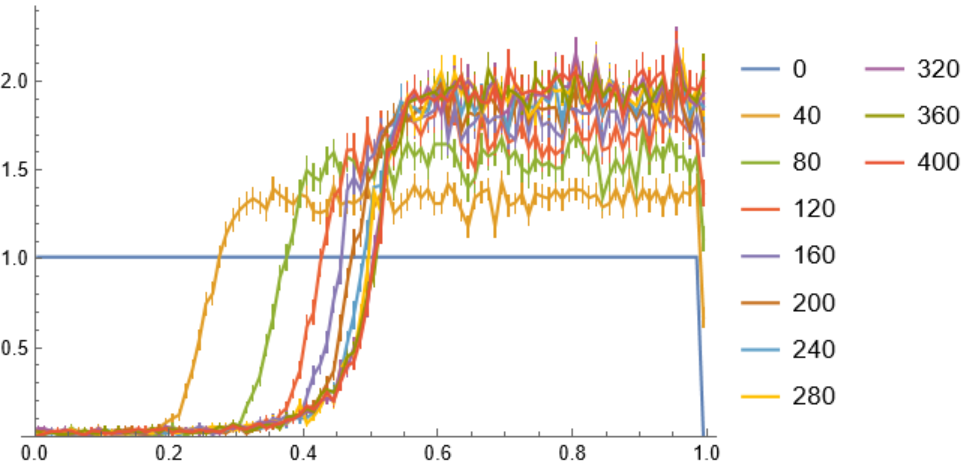Use more bins to get more detailed probability density functions:

 In:=Out=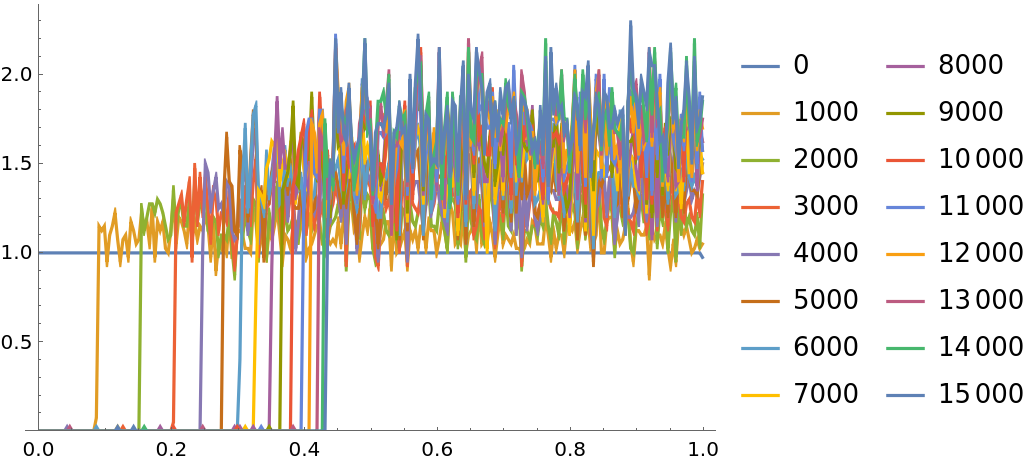### Options (7)

#### NewFitness (2)

Use a different fitness distribution for new agents:

 In:=Out=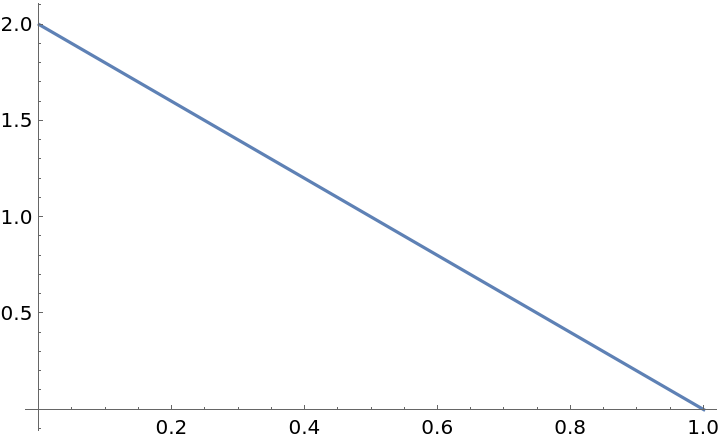Use this distribution:

 In:=Out=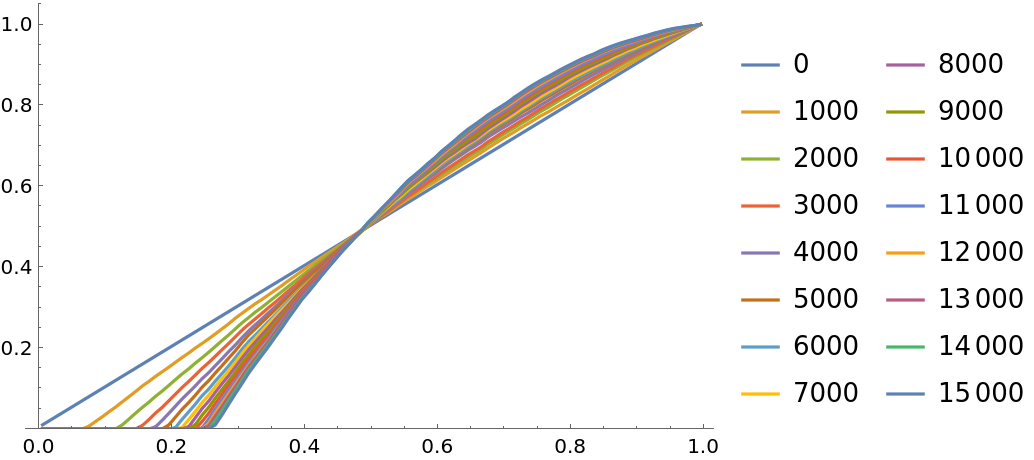Create agents with a normal distribution that is truncated such that the fitness is always between 0 and 1:

 In:=Out=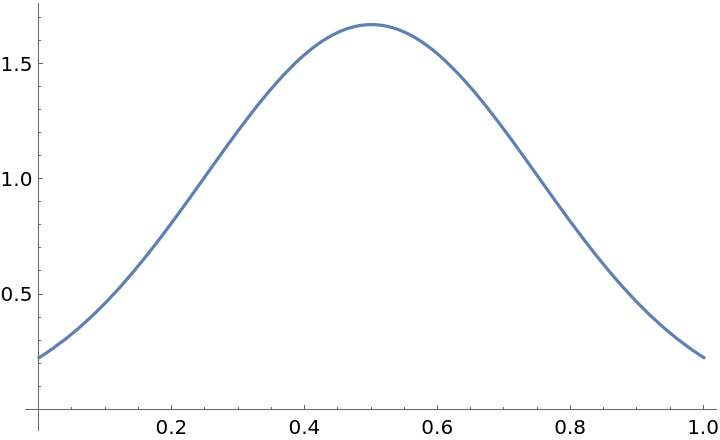Simulate with this fitness distribution for new agents:

 In:=Out=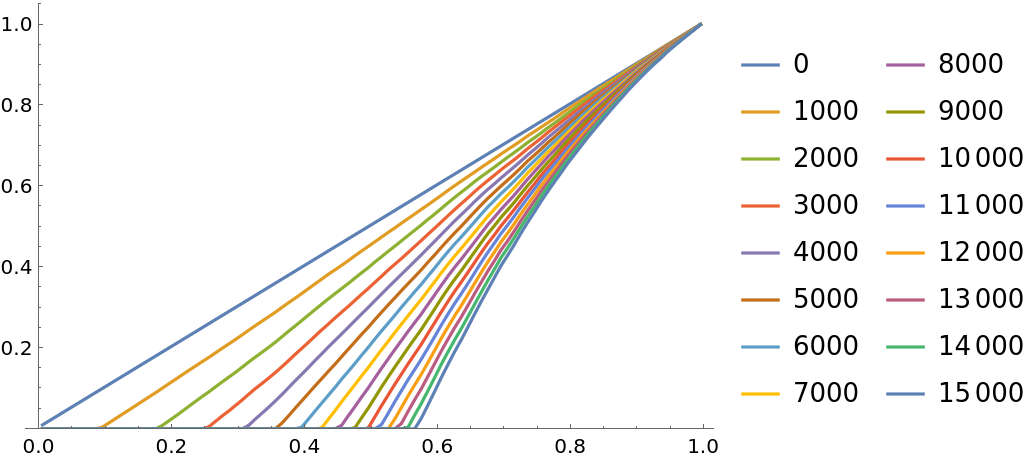#### Repetitions (2)

A single run of the simulation gives jagged results for the probability density function:

 In:=Out=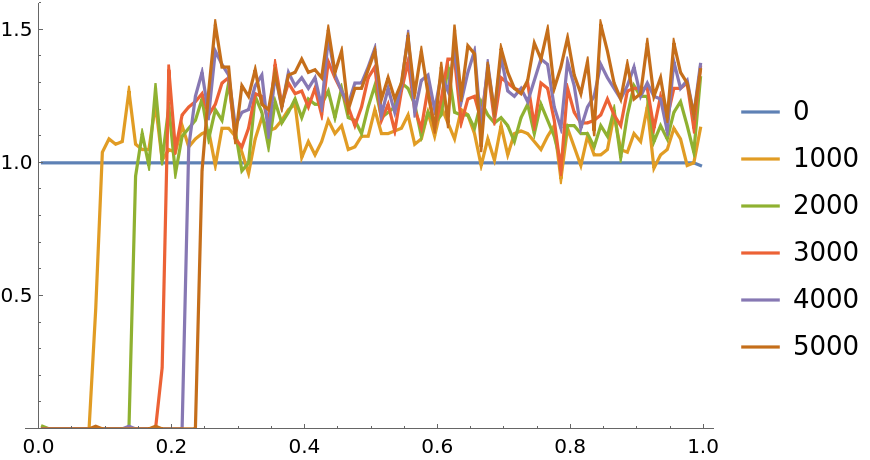Repeat the simulation and average:

 In:=Out=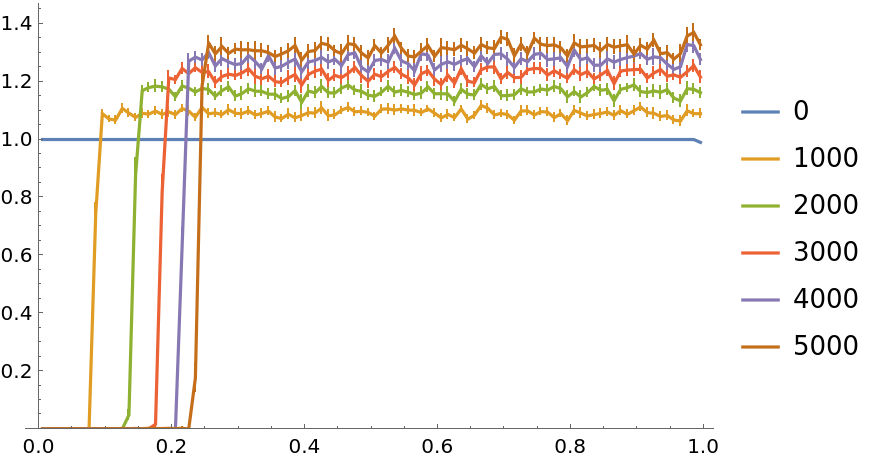#### Strengthening (3)

In case of survival of an agent, add 0.0001 to their fitness:

 In:=Out=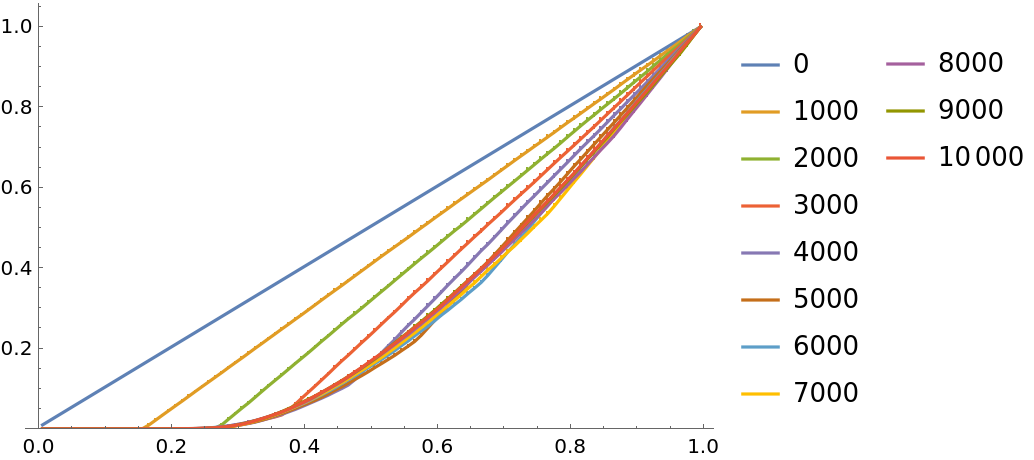In case of survival of an agent, multiply their fitness by 1.0003:

 In:=Out=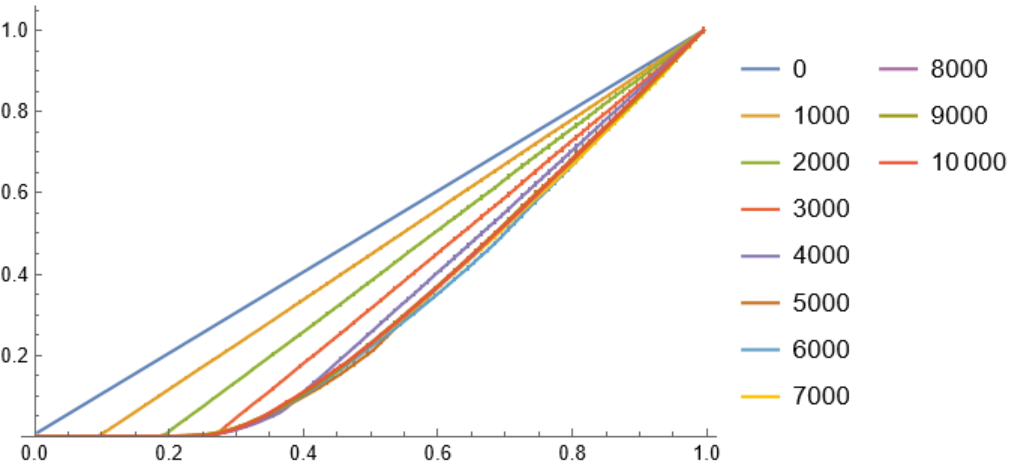In case of survival of an agent, improve their fitness by bring it 0.05% closer to 1:

 In:=Out=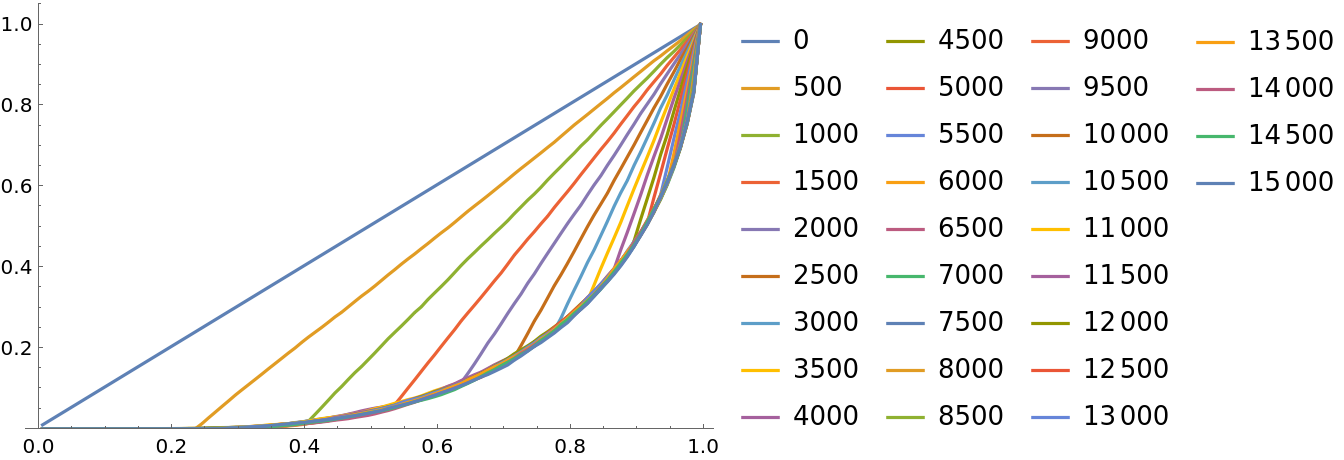### Neat Examples (3)

Find the mean strength of the agents:

 In:=Out=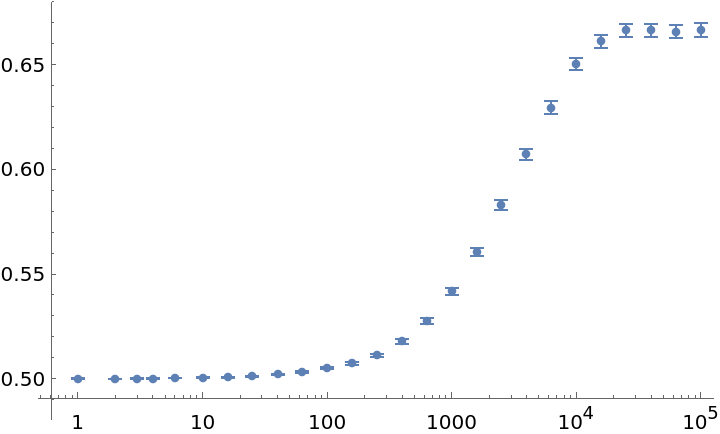Randomly remove agents based on a small chance:

 In:=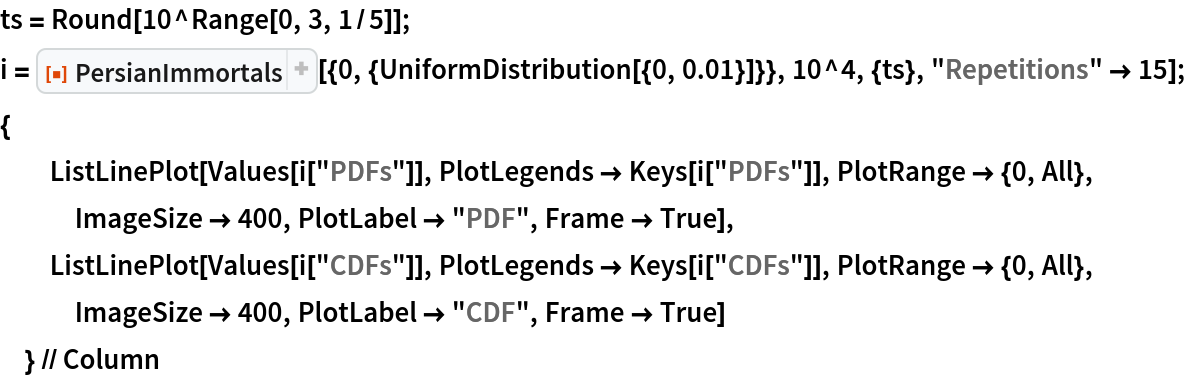Out=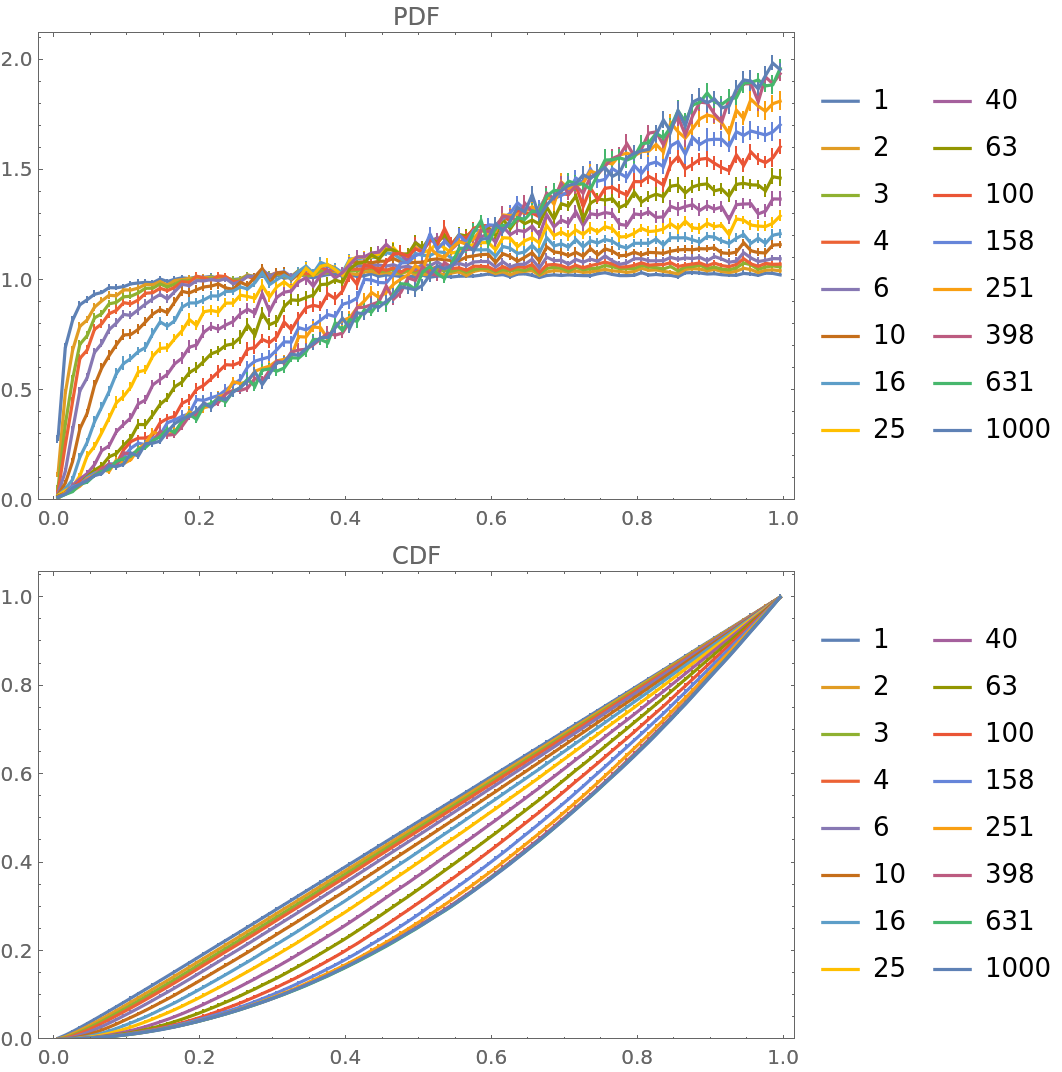Use a different fitness distribution for new agents:

 In:=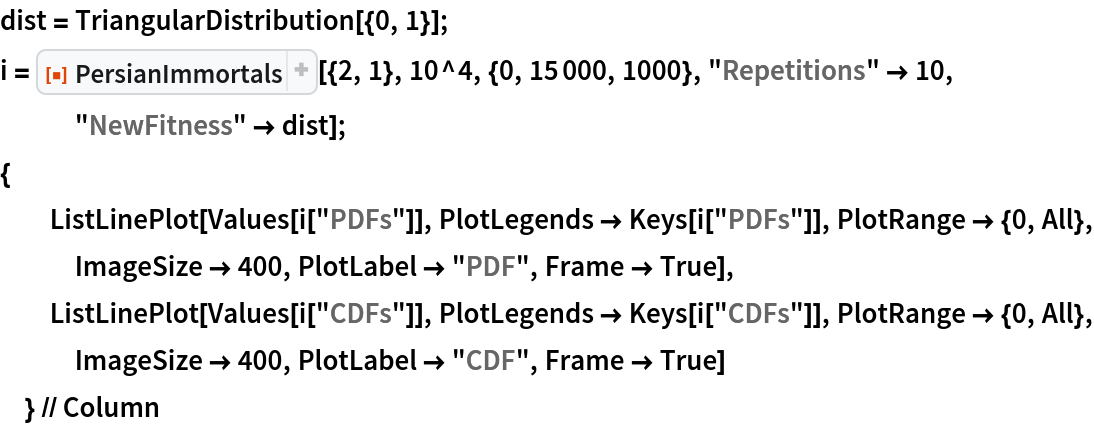Out=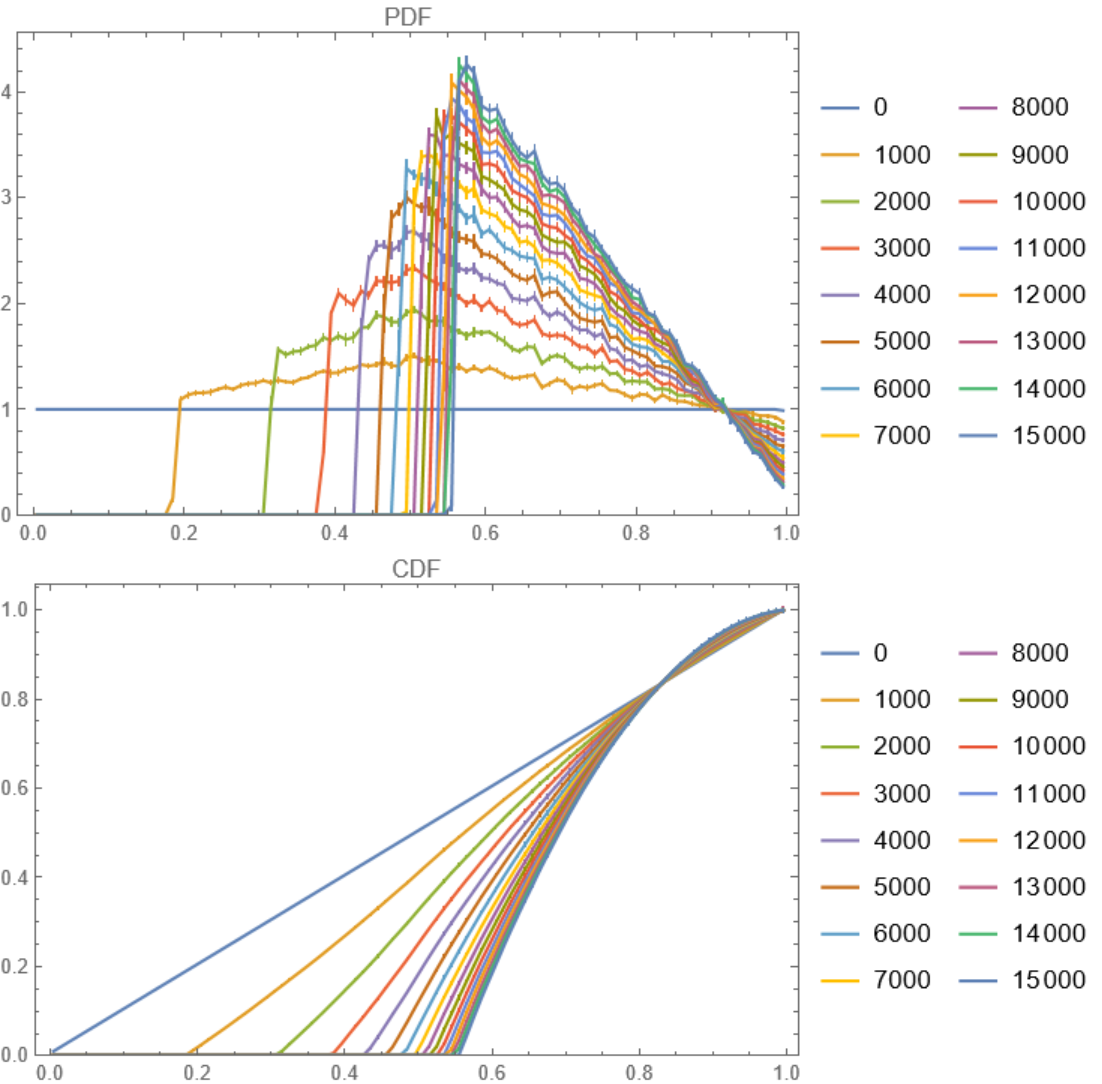SHuisman

## Version History

• 1.1.0 – 22 February 2023
• 1.0.0 – 13 February 2023